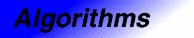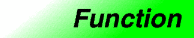Category: algorithms Component type: function

## Prototype

`Adjacent_difference` is an overloaded name; there are actually two `adjacent_difference` functions.

```template <class InputIterator, class OutputIterator>
OutputIterator result);

template <class InputIterator, class OutputIterator, class BinaryFunction>
OutputIterator result,
BinaryFunction binary_op);
```

## Description

`Adjacent_difference` calculates the differences of adjacent elements in the range `[first, last)`. This is, `*first` is assigned to `*result` , and, for each iterator `i` in the range `[first + 1, last)`, the difference of `*i` and `*(i - 1)` is assigned to `*(result + (i - first))`. 

The first version of `adjacent_difference` uses `operator-` to calculate differences, and the second version uses a user-supplied BinaryFunction. In the first version, for each iterator `i` in the range `[first + 1, last)`, `*i - *(i - 1)` is assigned to `*(result + (i - first))`. In the second version, the value that is assigned to `*(result + 1)` is instead `binary_op(*i, *(i - 1))`.

## Definition

Defined in the standard header numeric, and in the nonstandard backward-compatibility header algo.h.

## Requirements on types

For the first version:

• `ForwardIterator` is a model of ForwardIterator.
• `OutputIterator` is a model of OutputIterator.
• If `x` and `y` are objects of `ForwardIterator`'s value type, then `x - y` is defined.
• `InputIterator`s value type is convertible to a type in `OutputIterator`'s set of value types.
• The return type of `x - y` is convertible to a type in `OutputIterator`'s set of value types.

For the second version:

• `ForwardIterator` is a model of ForwardIterator.
• `OutputIterator` is a model of OutputIterator.
• `BinaryFunction` is a model of BinaryFunction.
• `InputIterator`'s value type is convertible to a `BinaryFunction`'s first argument type and second argument type.
• `InputIterator`s value type is convertible to a type in `OutputIterator`'s set of value types.
• `BinaryFunction`'s result type is convertible to a type in `OutputIterator`'s set of value types.

## Preconditions

• `[first, last)` is a valid range.
• `[result, result + (last - first))` is a valid range.

## Complexity

Linear. Zero applications of the binary operation if `[first, last)` is an empty range, otherwise exactly `(last - first) - 1` applications.

## Example

```int main()
{
int A[] = {1, 4, 9, 16, 25, 36, 49, 64, 81, 100};
const int N = sizeof(A) / sizeof(int);
int B[N];

cout << "A[]:         ";
copy(A, A + N, ostream_iterator<int>(cout, " "));
cout << endl;

cout << "Differences: ";
copy(B, B + N, ostream_iterator<int>(cout, " "));
cout << endl;

cout << "Reconstruct: ";
partial_sum(B, B + N, ostream_iterator<int>(cout, " "));
cout << endl;
}
```